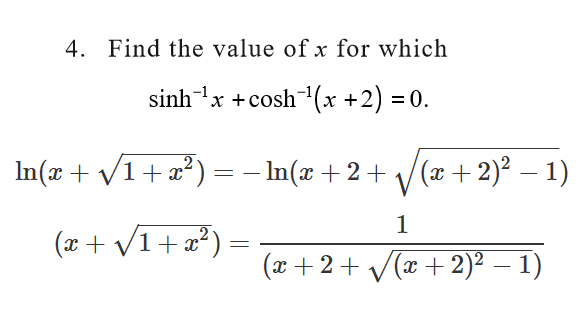# Hyperbolic function problem

## Homework Statement## The Attempt at a Solution

The attempt is in the picture. Is this the right method? Is there any faster method without cumbersome calculations?

Mark44
Mentor

## Homework Statement

View attachment 214910

## The Attempt at a Solution

The attempt is in the picture. Is this the right method? Is there any faster method without cumbersome calculations?
From this page, https://en.wikipedia.org/wiki/Inverse_hyperbolic_functions, I see that ##\sinh(\cosh^{-1}(x) = \sqrt{x^2 - 1}##, for |x| > 1.

•Clara Chung
From this page, https://en.wikipedia.org/wiki/Inverse_hyperbolic_functions, I see that ##\sinh(\cosh^{-1}(x) = \sqrt{x^2 - 1}##, for |x| > 1.
Thank you. I get the answer.
x=sinh(-arccosh(x+2))
=-sinh(arccosh(x+2))
=-root(x^2+4x+3)
And the website is very helpful

LCKurtz
•